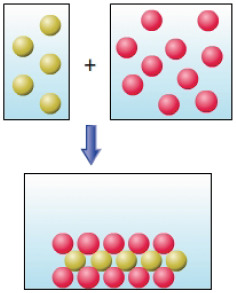# Problem: Part C. Consider the diagram. The red spheres represent the anion and the yellow spheres the cation. Which of the following combinations of reactants could the diagram represent?A. Pb2+(aq) + 2I−(aq) →B. 2Ag+(aq) + S2−(aq) →C. Mg2+(aq) + 2Cl−(aq) →D. 2NH4+(aq) + SO42−(aq) →Reactions in water solution can be classified based on the driving force that converts reactants to product. The three classes are precipitation reactions, acid-base neutralization reactions, and oxidation-reduction reactions.• In precipitation reactions a solid product forms from aqueous reactants. The product can be predicted by using solubility rules.• In acid-base neutralization reactions an acid (proton donor) reacts with a base (proton acceptor) to form an ionic salt and sometimes water. You can recognize this type of reaction by the presence of an acid and base in the reactants.• In oxidation-reduction reactions (or redox for short) electrons are transferred between reactants. So you will always see changes in oxidation number within this type of reaction.

###### FREE Expert Solution
90% (374 ratings)View Complete Written Solution
###### Problem Details

Part C. Consider the diagram. The red spheres represent the anion and the yellow spheres the cation. Which of the following combinations of reactants could the diagram represent?A. Pb2+(aq) + 2I(aq) →

B. 2Ag+(aq) + S2−(aq) →

C. Mg2+(aq) + 2Cl(aq) →

D. 2NH4+(aq) + SO42−(aq) →

Reactions in water solution can be classified based on the driving force that converts reactants to product. The three classes are precipitation reactions, acid-base neutralization reactions, and oxidation-reduction reactions.

• In precipitation reactions a solid product forms from aqueous reactants. The product can be predicted by using solubility rules.
• In acid-base neutralization reactions an acid (proton donor) reacts with a base (proton acceptor) to form an ionic salt and sometimes water. You can recognize this type of reaction by the presence of an acid and base in the reactants.
• In oxidation-reduction reactions (or redox for short) electrons are transferred between reactants. So you will always see changes in oxidation number within this type of reaction.